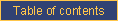homefeaturesDOCUMENTATIONpricingcontact ustechnical documentationCATI documentationcookbookrobotQuestionnaire structure

Tables

By default, CallWeb displays each question sequentially. The # TABLE instruction changes this default behaviour. When a series of variables belong to a matrix:

• the question text of the first variable is displayed;
• the note field of each question labels each row, and;
• the answer categories of the first question determine the columns labels and their order.

The syntax of the "# TABLE" instruction is:

# TABLE NAME = VAR1 - VAR2 HEADER=n PIXELS=n COLVARS=n POSITION=x FORCE_CODES TRANSPOSE SPECIAL=(n,...) CORNER=(...) VALIDATION

where

• NAME is the name of the matrix; any unique alphanumeric string is acceptable;
• VAR1 is the first variable to include in the table;
• VAR2 is the last variable to include in the table; obviously VAR2 must come after VAR1 in the questionnaire order; (the next arguments are all optional and their study should be reserved to more advanced users)
• HEADER=n is the frequency at which the column headings are repeated within the table, expressed in number of questions (-1 means no repetition); it can also be called using ENTETE=n
• PIXELS=n is the number of pixels the browser should attempt to give to each response column (-1 lets the browser figure out the layout);
• COLVARS=n is the number of variables to stack in the columns;
• the default is -1 which means that the columns of a table are defined by the first variable in the table set (it can also be called using the VARSCOL=n name)
• a value of 1 has a similar meaning except it directs the system to place the text of the first question within the matrix, above and across the answer categories
• a value greater than 1 means that the matrix displays that number of variables across the columns of the matrix — obviously, the number of variables in the matrix must be a multiple of the number of columns used across the columns; the system displays the first COLVARS columns across the first row of the matrix, then the second set of COLVARS columns across the second row, and so on; in the questionnaire .scw file, the variables must be ordered according to the sequence of use in the matrix, i.e., from left to right and from top to bottom (i.e., if the matrix comprises 8 questions (Q1-Q8) and is defined as using two variables across the columns, Q1 and Q2 are displayed in that order on the first row, then Q3 and Q4 on the second row, etc.)
• POSITION=x determines the position of the table on the page: "LEFT" or "GAUCHE" puts the table on the left margin; "RIGHT" or "DROITE" does the same on the right margin; "CENTER" or "CENTRE" centres the table horizontally on the page. The default value is LEFT.
• FORCE_CODES is a keyword which forces the variables in the matrix to use their own response codes (whereas, without it, every variable in the matrix uses the codes of the first-row variable for their column order); this cannot be used along with permutated response codes; for example, this can be used to display a drop-down list of provinces and territories in a table that otherwise contains single boxes of text;
• TRANSPOSE reverses the presentation of rows and columns. By default, CallWeb displays the response categories as columns and the questions as rows such that one (or more) selection is expected on each row; with TRANSPOSE, the questions are arrayed as columns and the response codes make up the rows such that one (or more) response is expected for each column. For example, parent educational achievement could be requested with each of the mother and the father occupying one column of the matrix;
• SPECIAL is an instruction which identifies the response codes that are to be coloured differently from the normal table colour; the normal colour is driven by the # M_COULEURCELLULES instruction while the SPECIAL codes are painted with the # M_COULEURSPECIALE instruction; the column header cell, which is normally coloured by the # M_COULEURTITRESCOLONNES instruction, also bears the special colour for the selected codes. All vertical variables of the table share these special codes such that, if code 9 is to be special-coloured, all codes 9, in all vertical variables, are coloured as such; also refer to the "S" answer category behaviour code;
• CORNER is text within parentheses that appears in the upper left hand side corner of the matrix; language codes in the form of [xx] may be embedded within this text; if the text includes parentheses, insert them as &# codes where an open parenthesis is the code &#40; and a closed parenthesis is the code &#41;
• VALIDATION is a special validation instruction for the matrix as a whole; validation instructions include:
• EXCLUSIF(min,max,A)
this validation instruction demands that the respondent provides only one answer per column of the table; the system accepts a minimum of MIN answers between the values of 1 and MAX; if the "A" argument is provided, the test is performed on the open end parts of the questions in the matrix; otherwise it is performed on the normal questions.
• ORDRE(min,max,A)
this validation instruction places demands on the respondent similar to EXCLUSIF except the answers are interpreted as an order of preference; therefore, the set of answers must include, at a minimum, all values between 1 and the MIN argument out of a maximum of MAX answers.

Important note: by definition, tables are displayed within pages; therefore, the questions making up the matrix must also be part of a (and the same) page defined with a # GROUP instruction.

The following statements show an example which incorporates all of the circumstances described in this section:

# Group Alpha = Q1-Q10
# Table Beta = Q2-Q8
# Permutation Gamma = Q3-Q7e-mail technical support!